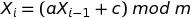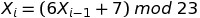## A Linear Congruential Generator (LCG) in R

In my simulation classes, we talk about how to generate random numbers. One of the techniques we talk about is the Linear Congruential Generator (LCG). Starting with a seed, the LCG produces the first number in the sequence, and then uses that value to generate the second one. The second value is used to generate the third, the third to generate the fourth, and so on. The equation looks like this:where a is a multiplier, c is a shift, and m is a modulus. (Modulus just means “find me the remainder when you divide the stuff to the left of the mod operator by the stuff to the right”. Fortunately there is an easy way to do this in R: a mod b is expressed as a %% b.) When you use the LCG to generate a stream of random numbers, they will always be between 0 and (m-1), and the sequence will repeat every m occurrences. When you take the sequence of values x and divide it by the mod m, you get uniformly distributed random numbers between 0 and 1 (but never quite hitting 1). The LCG is a pseudorandom number generator because after a while, the sequence in the stream of numbers will begin to repeat.

I wrote an exam last week with some LCG computations on it, but then I got lazy and didn’t want to do the manual calculations… so I wrote a function in R. Here it is. It returns two different vectors: the collection of numbers between 0 and (m – 1) that the LCG produces, and the collection of uniformly distributed random numbers between 0 and 1 that we get by dividing the first collection by m:

```lcg <- function(a,c,m,run.length,seed) {
x <- rep(0,run.length)
x <- seed
for (i in 1:(run.length-1)) {
x[i+1] <- (a * x[i] + c) %% m
}
U <- x/m # scale all of the x's to
# produce uniformly distributed
# random numbers between [0,1)
return(list(x=x,U=U))
}```

So for example, if your LCG looks like this:Then, after loading in the function above, you could choose a seed (say, 5) and generate a stream of 20 random numbers:

```> z <- lcg(6,7,23,20,5)
> z
\$x
 5 14 22 1 13 16 11 4 8 9 15 5 14 22 1 13 16 11
 4 8

\$U
 0.21739130 0.60869565 0.95652174 0.04347826 0.56521739
 0.69565217 0.47826087 0.17391304 0.34782609 0.39130435
 0.65217391 0.21739130 0.60869565 0.95652174 0.04347826
 0.56521739 0.69565217 0.47826087 0.17391304 0.34782609```

The values contained within z\$x are the numbers that the LCG produces, and the values contained within z\$U are the values of z\$x scaled to fall between 0 and 1. Both z\$x and z\$U are uniformly distributed, although the pattern will become more apparent as you generate more and more random numbers in your stream. Here’s what they look like with 10000 numbers in the stream:

```> z <- lcg(6,7,23,10000,5)
> par(mfrow=c(1,2))
> hist(z\$x,col="red")
> hist(z\$U,col="purple")```But how good is your random number generator? First, you know you want a large (and preferably odd valued mod). That way, the numbers will not repeat so quickly. But there are also considerations dealing with what multiplier and shift you should use. I like checking out the quality of my random number generator by plotting how they move in sequence around a two dimensional grid: the better the random number generator, the more filling of the space you will see.

You can play around with these plots to find a “good” random number generator:

```> z1 <- lcg(6,7,23,2000,5) # "Bad" LCG
> z2 <- lcg(1093,18257,86436,2000,12) # "Good" LCG
> par(mfrow=c(1,2))
> plot( z1\$U[1:(length(z1\$U)-1)], z1\$U[2:length(z1\$U)] , main="Bad")
> plot( z2\$U[1:(length(z2\$U)-1)], z2\$U[2:length(z2\$U)] , main="Good")```Although there are many heuristics you can use to avoid creating a “bad” random number generator, it’s really difficult to design a “good” one. People make careers out of this. I don’t claim to be an expert, I just know how to test to see if a random number generator is suitable for my simulations. (I also really like the handout at http://www.sci.csueastbay.edu/~btrumbo/Stat3401/Hand3401/CongGenIntroB.pdf that explains this graphing approach more, and also talks about how you have to look at patterns in three dimensions as well. When I was searching around for a “good LCG that would give me a nice graph” I found this handout.)

• Mark FergusonBe very wary of cooking up your own RNG. It’s certainly fun and interesting to understand the machinery behind them, but unless you are really focused on that subject you are more likely to get into trouble than anything else.

In terms of examining the performance of RNGs, I highly recommend looking into the DIEHARD battery of tests, which originated with George Marsaglia.

One last point: often you don’t need a (quasi)random sequence. If you are interested in path dependent simulations, then yes, those simulations are going to need qRNGs. If you are simply evaluating an expectation, your best friend is a “low discrepancy” equidistributed sequence. These are sequences that essentially fill space efficiently – the next number in the sequence is “as far away” as possible from the other numbers already delivered in the sequence. Use these to fill a unit hypercube as needed, and invert through your distribution function as you would with qRNGs. See Wikipedia for an overview and references.Agreed… and like I mentioned, I’m not an expert on RNG, just someone who sometimes needs to generate random numbers. I think the LCG case is pretty instructive as a teaching tool though — helps students understand the most salient points underlying RNG (and why you might want – or not want – to do it in the first place). Thanks for the very important reminder to everyone!!

•Nice post,
I was trying to calculate manually the numbers generated but I don’t understand where the second element,14, is coming from. In my understanding the calculation would be 6*(5+7) mod 23 or 72 mod 23 and I don’t get 14.
What am I doing wrong?Hi Ruben — the issue was the parentheses. The right images for the equations are up now… check that and you will get a 14 for your x 🙂

• Pingback: Distilled News | Data Analytics & R

•Hi Nicole, do I need to load any specific package in R for the LCG ? Thanks